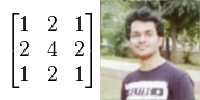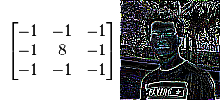### Kernel Image Processing : Image Filters (with Java Code)

In image processing a kernel is a small matrix which is used to perform operations like blurring, sharpening, edge-detection etc. on images. The operation is performed by convolution between the image's 2D pixel matrix and the 2D kernel matrix. Convolution is a mathematical operation between two functions to produce a third function which may be defined as a distorted version of the two input functions.Convolution of two functions (Source: Wikipedia)

The Kernel Matrix:

a) It should be a small odd order 2D matrix like 3x3, 5x5 etc. Odd order is required because the matrix should have a center element.
b) The sum of the elements of the matrix should be 1 to get the output image's brightness equal to input image's. A sum greater than 1 increases the brightness while a sum less than 1 decreases it.

The Convolution:

Imagine the image as a large 2D matrix and the smaller kernel matrix (say 3x3) overlapping the image from the zeroth pixel with the kernel matrix's center element situated at 0,0 pixel of the image in the beginning. Then the kernel matrix slides over the image matrix and each kernel element is multiplied by the underlying pixel. Hence for getting the output pixel of a single input pixel multiplication of total 9 pixels (in case of 3x3 matrix) with the corresponding kernel matrix elements is performed with the current pixel being multiplied by the center element of the matrix. The output pixel is equal to the sum of results of the nine multiplications.

As you may have guessed by now we are in a problem here because when we go near the edges of the image our kernel matrix goes out of the image's matrix. We can either solve this problem by crop or wrap. In case of crop we simply neglect those pixels and hence the output image is a bit cropped while in wrap we assume the image to be wrapped i.e. the pixel value is taken from the opposite edge.

If the computed value goes below 0 or above 255 the value is truncated to 0 or 255 respectively.

The Pseudo Code:

```for each row in image:
for each pixel in row:
set sum = 0
for each row in kernel:
for each element in kernel row:
multiply element value by corresponding pixel value

set output image pixel to value of sum
```

The Java Code:

```// ConvolutionFilter.java
// Author: Abdul Fatir
// E-Mail: abdulfatirs@gmail.com

import javax.imageio.ImageIO;
import java.awt.image.BufferedImage;
import java.awt.Color;
import java.io.File;
import java.io.IOException;
import static java.lang.System.out;
public class ConvolutionFilter
{
public static String filename = "raw.png";
public static void main(String args[])throws IOException
{

out.print("Kernel Order:");

float[][] kernel = new float[order][order];
float sum_of_elements = 0.0f;
float mult_factor = 1.0f;
float bias = 0f;

// Getting the Kernel Matrix as input from the user
for(int i=0; i < order; i++)
for(int j=0; j < order; j++)
{
out.print(i+","+j+":");
}

out.println("\nThe Kernel Matrix is:\n");

for(int i=0; i < order; i++)
{
for(int j=0; j < order; j++)
{
out.print("\t"+kernel[i][j]);
sum_of_elements += kernel[i][j];
}
out.println();
}

out.println("\nThe sum of matrix elements is: "+sum_of_elements);

// mult_factor is the value with which each computed sum is multiplied
// mult_factor = 1 gives no changes to input kernel matrix
out.print("\nMultiplication Factor: ");

// Bias can be added to increase brightness of the image
// bias = 0 gives no change in brightness if the sum_of_elements is 1
out.print("Bias: ");

BufferedImage input,output;

int WIDTH = input.getWidth();
int HEIGHT = input.getHeight();

output = new BufferedImage(WIDTH, HEIGHT, input.getType());
out.println("[*] Rendering the image...");
for(int x=0;x<WIDTH;x++)
{
for(int y=0;y<HEIGHT;y++)
{
float red=0f,green=0f,blue=0f;
for(int i=0;i<order;i++)
{
for(int j=0;j<order;j++)
{
// Calculating X and Y coordinates of the pixel to be multiplied with current kernel element
// In case of edges of image the '% WIDTH' wraps the image and the pixel from opposite edge is used
int imageX = (x - order / 2 + i + WIDTH) % WIDTH;
int imageY = (y - order / 2 + j + HEIGHT) % HEIGHT;

int RGB = input.getRGB(imageX,imageY);
int R = (RGB >> 16) & 0xff; // Red Value
int G = (RGB >> 8) & 0xff;	// Green Value
int B = (RGB) & 0xff;		// Blue Value

// The RGB is multiplied with current kernel element and added on to the variables red, blue and green
red += (R*kernel[i][j]);
green += (G*kernel[i][j]);
blue += (B*kernel[i][j]);
}
}
int outR, outG, outB;
// The value is truncated to 0 and 255 if it goes beyond
outR = Math.min(Math.max((int)(red*mult_factor),0),255);
outG = Math.min(Math.max((int)(green*mult_factor),0),255);
outB = Math.min(Math.max((int)(blue*mult_factor),0),255);
// Pixel is written to output image
output.setRGB(x,y,new Color(outR,outG,outB).getRGB());
}
}
out.print("[?] Save file as:");
ImageIO.write(output,"PNG", new File(outputfname+".png"));
out.print("[+] File saved as "+outputfname+".png");
}

}
```

Example input matrices and corresponding effects:

1) Original Image
Factor = 1, Bias = 0The Original Matrix & Image

2) Sharpened Image
Factor = 1, Bias = 0Sharpening Matrix & Image
3) Blurred Image
Factor = 1/16 = 0.0625, Bias = 0Blur Matrix & Image
4) Edge Detect
Factor = 1, Bias = 0Edge Detection Matrix & Image

5) Motion Blur
Factor = 1/5 = 0.2, Bias = 0Motion Blur Matrix & Image
6) Emboss
Factor = 1, Bias =128Emboss Matrix & Image

References:
 lodev.org/cgtutor/filtering.html
 http://en.wikipedia.org/wiki/Kernel_(image_processing)
 http://en.wikipedia.org/wiki/Convolution